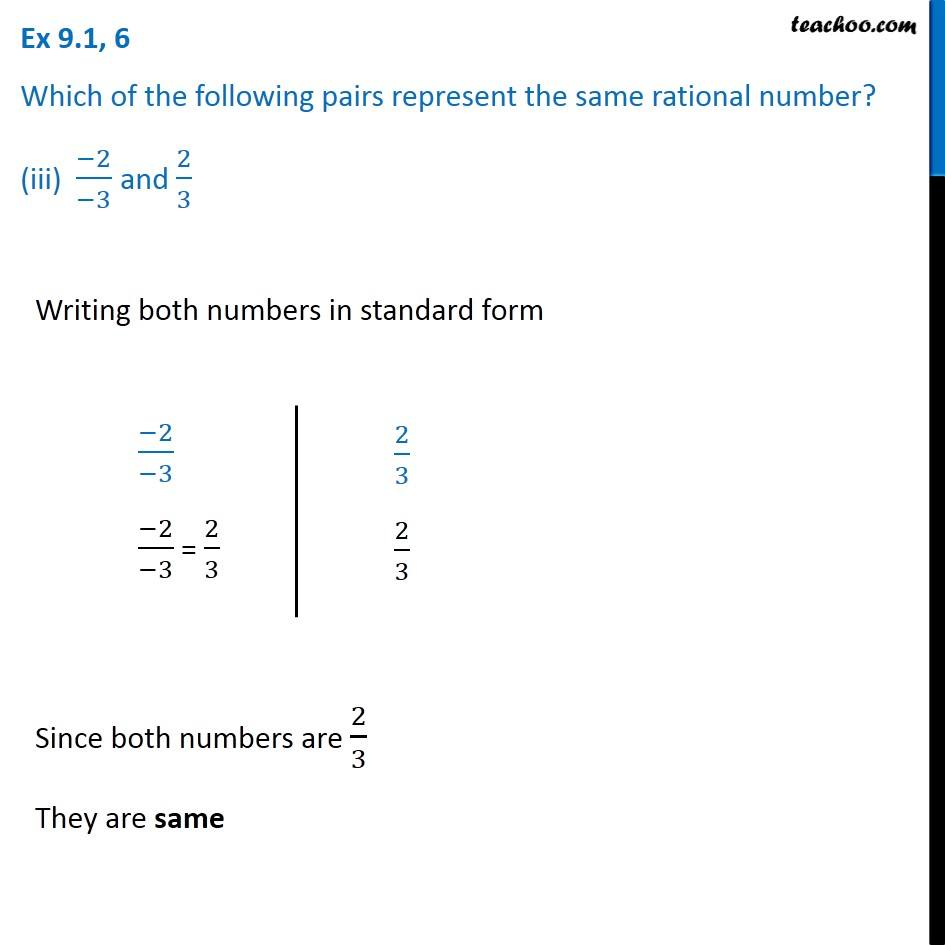Ex 9.1

Chapter 9 Class 7 Rational Numbers
Serial order wise### Transcript

Ex 9.1, 6 Which of the following pairs represent the same rational number? (iii) (−2)/(−3) and 2/3 Writing both numbers in standard form (−2)/(−3) (−2)/(−3) = 2/3 2/3 2/3 Since both numbers are 2/3 They are same